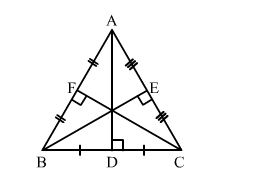# ∆ABC is an equilateral triangle of a side 2a units.

Question:

ABC is an equilateral triangle of a side 2a units. Find each of its altitudes.

Solution:Let AD, BE and CF be the altitudes of ∆ABC meeting BC, AC and AB at D, E and F, respectively.
Then, D, E and F are the midpoints of BC, AC and AB, respectively.

In right-angled ∆ABD, we have:
AB = 2a and BD = a
Applying Pythagoras theorem, we get:

$A B^{2}=A D^{2}+B D^{2}$

$A D^{2}=A B^{2}-B D^{2}=(2 a)^{2}-a^{2}$

$A D^{2}=4 a^{2}-a^{2}=3 a^{2}$

$A D=\sqrt{3} a$ units

Similarly,

$\mathrm{BE}=a \sqrt{3}$ units and $\mathrm{CF}=a \sqrt{3}$ units